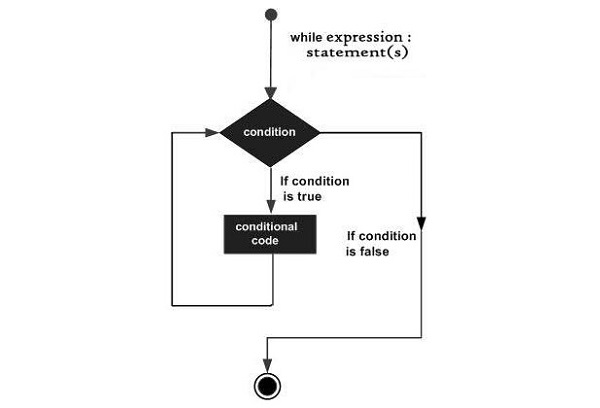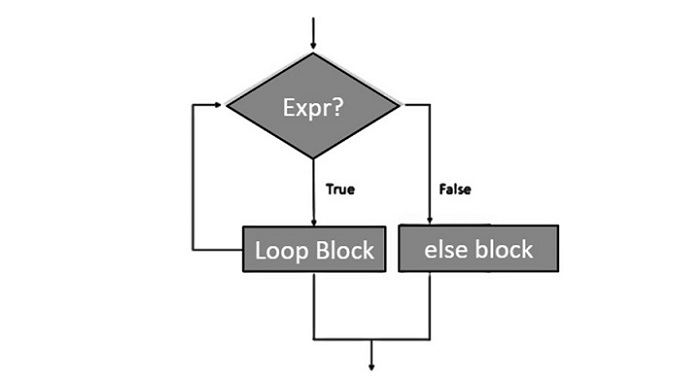# Python - The while Loop

Normally, flow of execution of steps in a computer program goe from start to end. However, instead of the next step, if the flow is redirected towards any earlier step, it constitutes a loop.

A while loop statement in Python programming language repeatedly executes a target statement as long as a given boolean expression is true.

### Syntax

The syntax of a while loop in Python programming language is −

```while expression:
statement(s)
```

The while keyword is followed by a boolean expression, and then by colon symbol, to start an indented block of statements. Here, statement(s) may be a single statement or a block of statements with uniform indent. The condition may be any expression, and true is any non-zero value. The loop iterates while the boolean expression is true.

As soon as the expression becomes false, the program control passes to the line immediately following the loop.

If it fails to turn false, the loop continues to run, and doesnâ€™t stop unless forcefully stopped. Such a loop is called infinite loop, which is undesired in a computer program.

The following flow diagram illustrates the while loop −### Example 1

In Python, all the statements indented by the same number of character spaces after a programming construct are considered to be part of a single block of code. Python uses indentation as its method of grouping statements.

```count=0
while count<5:
count+=1
print ("Iteration no. {}".format(count))

print ("End of while loop")
```

We initialize count variable to 0, and the loop runs till "count<5". In each iteration, count is incremented and checked. If itâ€™s not 5 next repetion takes place. Inside the looping block, instantenous value of count is printed. When the while condition becomes false, the loop terminates, and next statement is executed, here it is End of while loop message.

### Output

On executing, this code will produce the following output −

```Iteration no. 1
Iteration no. 2
Iteration no. 3
Iteration no. 4
Iteration no. 5
End of while loop
```

### Example 2

Here is another example of using the while loop. For each iteration, the program asks for user input and keeps repeating till the user inputs a non-numeric string. The isnumeric() function that returns true if input is an integer, false otherwise.

```var='0'
while var.isnumeric()==True:
var=input('enter a number..')
if var.isnumeric()==True:
print ("End of while loop")
```

### Output

On executing, this code will produce the following output −

```enter a number..10
enter a number..100
enter a number..543
enter a number..qwer
End of while loop
```

## The Infinite Loop

A loop becomes infinite loop if a condition never becomes FALSE. You must be cautious when using while loops because of the possibility that this condition never resolves to a FALSE value. This results in a loop that never ends. Such a loop is called an infinite loop.

An infinite loop might be useful in client/server programming where the server needs to run continuously so that client programs can communicate with it as and when required.

### Example 3

Let's take an example to understand how the infinite loop works in Python −

```#!/usr/bin/python3
var = 1
while var == 1 : # This constructs an infinite loop
num = int(input("Enter a number :"))
print ("You entered: ", num)
print ("Good bye!")
```

### Output

On executing, this code will produce the following output −

```Enter a number :20
You entered: 20
Enter a number :29
You entered: 29
Enter a number :3
You entered: 3
Enter a number :11
You entered: 11
Enter a number :22
You entered: 22
Enter a number :Traceback (most recent call last):
File "examples\test.py", line 5, in
num = int(input("Enter a number :"))
KeyboardInterrupt
```

The above example goes in an infinite loop and you need to use CTRL+C to exit the program.

## The while-else Loop

Python supports having an else statement associated with a while loop statement.

If the else statement is used with a while loop, the else statement is executed when the condition becomes false before the control shifts to the main line of execution.

The following flow diagram shows how to use else with while statement −### Example

The following example illustrates the combination of an else statement with a while statement. Till the count is less than 5, the iteration count is printed. As it becomes 5, the print statement in else block is executed, before the control is passed to the next statement in the main program.

```count=0
while count<5:
count+=1
print ("Iteration no. {}".format(count))
else:
print ("While loop over. Now in else block")
print ("End of while loop")
```

### Output

On executing, this code will produce the following output

```Iteration no. 1
Iteration no. 2
Iteration no. 3
Iteration no. 4
Iteration no. 5
While loop over. Now in else block
End of while loop
```